# 聚类算法之Label Propagation钱魏Way · · 5 次浏览

## 标签传播算法（label propagation）简介

### 半监督学习

• 监督学习可以认为是我们有非常多的labeled标注数据来train一个模型，期待这个模型能学习到数据的分布，以期对未来没有见到的样本做预测。
• 非监督学习就是没有任何的labeled数据，就是平时所说的聚类，利用他们本身的数据分布，给他们划分类别。
• 半监督学习，顾名思义就是处于两者之间的，只有少量的labeled数据，我们试图从这少量的labeled数据和大量的unlabeled数据中学习到有用的信息。

• Smoothness平滑假设：相似的数据具有相同的label。
• Cluster聚类假设：处于同一个聚类下的数据具有相同label。
• Manifold流形假设：处于同一流形结构下的数据具有相同label。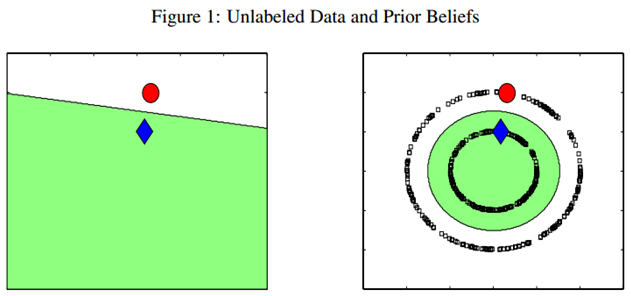### 标签传播算法（label propagation）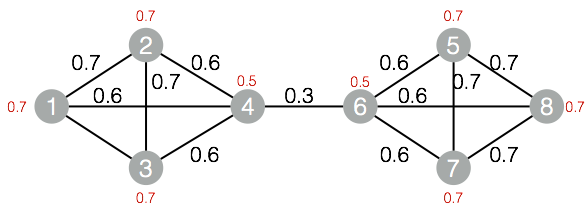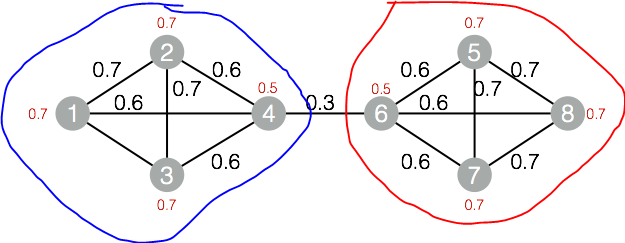LPA算法优点

Label Propagation 算法最大的优点是其算法逻辑比较简单，相比于优化模块度的过程，算法速度非常快。Label Propagation算法利用网络自身的结构指导标签的传播过程，在这个过程中无需优化任何函数。在算法开始前我们不必知道社区的个数，随着算法的迭代，在最终的过程中，算法将自己决定社区的个数。

LPA算法缺点

• 更新顺序：节点标签更新顺序随机，但是很明显，越重要的节点越早更新会加速收敛过程
• 随机选择：如果一个节点的出现次数最大的邻居标签不止一个时，随机选择一个标签作为自己标签。这种随机性可能会带来一个雪崩效应，即刚开始一个小小的聚类错误会不断被放大。不过话也说话来，如果相似邻居节点出现多个，可能是weight计算的逻辑有问题，需要回过头去优化weight抽象和计算逻辑

## 标签传播算法（label propagation）算法原理

Label Propagation 算法是一种基于标签传播的局部社区划分算法。对于网络中的每一个节点，在初始阶段，Label Propagation 算法对每个节点初始化一个唯一的标签，在每次的迭代过程中，每个节点根据与其相连的节点所属的标签改变自己的标签，更改的原则是选择与其相连的节点中所属标签最多的社区标签为自己的社区标签，这便是标签传播的含义。随着社区标签不断传播，最终，连接紧密的节点将有共同的标签。

Label Propagation算法包括两大步骤：1）构造相似矩阵；2）标签传播。

### 构建相似矩阵

LP算法是基于Graph的，因此我们需要先构建一个图。我们为所有的数据构建一个图，图的节点就是一个数据点，包含labeled和unlabeled的数据。节点i和节点j的边表示他们的相似度。这个图的构建方法有很多，这里我们假设这个图是全连接的，节点i和节点j的边权重为：

$$w_{ij}=\exp(-\frac{\left \| x_i-x_j\right \|^2}{\alpha ^2})$$

### 标签传播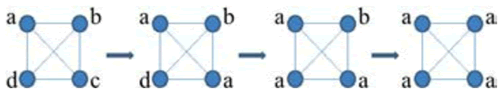$$C_x(t)=f(C_{x_1}(t-1),C_{x_2}(t-1),….C_{x_k}(t-1))$$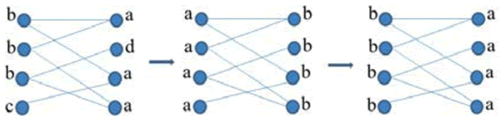$$C_x(t)=f(C_{x_{i1}}(t),…,C_{x_{im}}(t),C_{x_{i(m+1)}}(t-1),…,C_{x_{ik}}(t-1))$$

## 标签传播算法（label propagation）的Python实现

def label_propagation(vector_dict, edge_dict):
'''标签传播
input:  vector_dict(dict)节点：社区
edge_dict(dict)存储节点之间的边和权重
output: vector_dict(dict)节点：社区
'''
# 初始化，设置每个节点属于不同的社区
t = 0
# 以随机的次序处理每个节点
while True:
if (check(vector_dict, edge_dict) == 0):
t = t + 1
print("iteration: ", t)
# 对每一个node进行更新
for node in vector_dict.keys():
print(vector_dict)
else:
break
return vector_dict

'''得到相邻接的节点中标签数最多的标签
input:  vector_dict(dict)节点：社区
output: 节点所属的社区
'''
label_dict = {}
node_id_weight = node.strip().split(":")
node_id = node_id_weight  # 邻接节点
node_weight = int(node_id_weight)  # 与邻接节点之间的权重
if vector_dict[node_id] not in label_dict:
label_dict[vector_dict[node_id]] = node_weight
else:
label_dict[vector_dict[node_id]] += node_weight

# 找到最大的标签
sort_list = sorted(label_dict.items(), key=lambda d: d, reverse=True)
return sort_list

def check(vector_dict, edge_dict):
'''检查是否满足终止条件
input:  vector_dict(dict)节点：社区
edge_dict(dict)存储节点之间的边和权重
output: 是否需要更新
'''
for node in vector_dict.keys():
node_label = vector_dict[node]  # 节点node所属社区
if node_label == label:  # 对每个节点，其所属的社区标签是最大的
continue
else:
return 0
return 1

f = open(filePath)
vector_dict = {}
edge_dict = {}
lines = line.strip().split("   ")
for i in range(2):
if lines[i] not in vector_dict:
vector_dict[lines[i]] = int(lines[i])
edge_list = []
if len(lines) == 3:
edge_list.append(lines[1 - i] + ":" + lines)
else:
edge_list.append(lines[1 - i] + ":" + "1")
edge_dict[lines[i]] = edge_list
else:
edge_list = edge_dict[lines[i]]
if len(lines) == 3:
edge_list.append(lines[1 - i] + ":" + lines)
else:
edge_list.append(lines[1 - i] + ":" + "1")
edge_dict[lines[i]] = edge_list
return vector_dict, edge_dict

if __name__ == '__main__':
filePath = './data/label_data.txt'
print(vector)
print(edge)
vector_dict = label_propagation(vector, edge)
print(vector_dict)
cluster_group = dict()
for node in vector_dict.keys():
cluster_id = vector_dict[node]
print("cluster_id, node", cluster_id, node)
if cluster_id not in cluster_group.keys():
cluster_group[cluster_id] = [node]
else:
cluster_group[cluster_id].append(node)
print(cluster_group)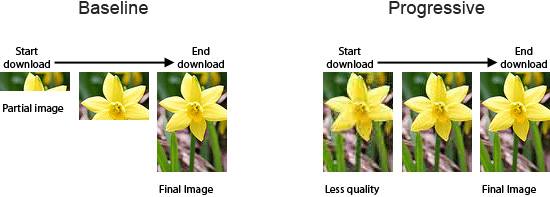##### 使用渐进式JPEG来提升用户体验##### 网站开发图片格式对比与选择